Open in App
Not now

# What is Conditional Programming in Scratch?

• Last Updated : 07 Aug, 2021

Scratch is a high-level visual programming language tool that interacts with users through diagrams and blocks that have the basics of a program inbuilt in it. Scratch is used to make interactive programs especially for kids using the block kind of interfaces so that they can easily learn languages and it is free to use. In this article, we will learn about coordinates and conditionals.

### Coordinates System

In Scratch, the coordinate system is used to locate the sprite(actor if the scene) on the screen or we can say that the coordinate system is a mathematical grid with infinite values. It is a two-dimensional cartesian coordinate system and is used to allocate a point/object or to extract the position of the object/point.

Position: Coordinate system has two coordinates, i.e., X position and Y position to find the position of the sprite on the screen. Here, X position denotes the horizontal location of the sprite and the Y position denotes the vertical location of the sprite. The X position can range from 240 to -240, where 240 is the rightmost side of the stage or screen and -240 is the leftmost, and the Y position ranges from 180 to -180, where 180 is the topmost side and -180 is the bottom-most side. and coordinates can be written as (X, Y). For example, (3, 4) is the coordinate of a sprite on the stage.

To change the backdrop of the scratch to a xy-grid. In the bottom right corner of the screen, click on the “backdrop” option to choose the x-y coordinate system as background.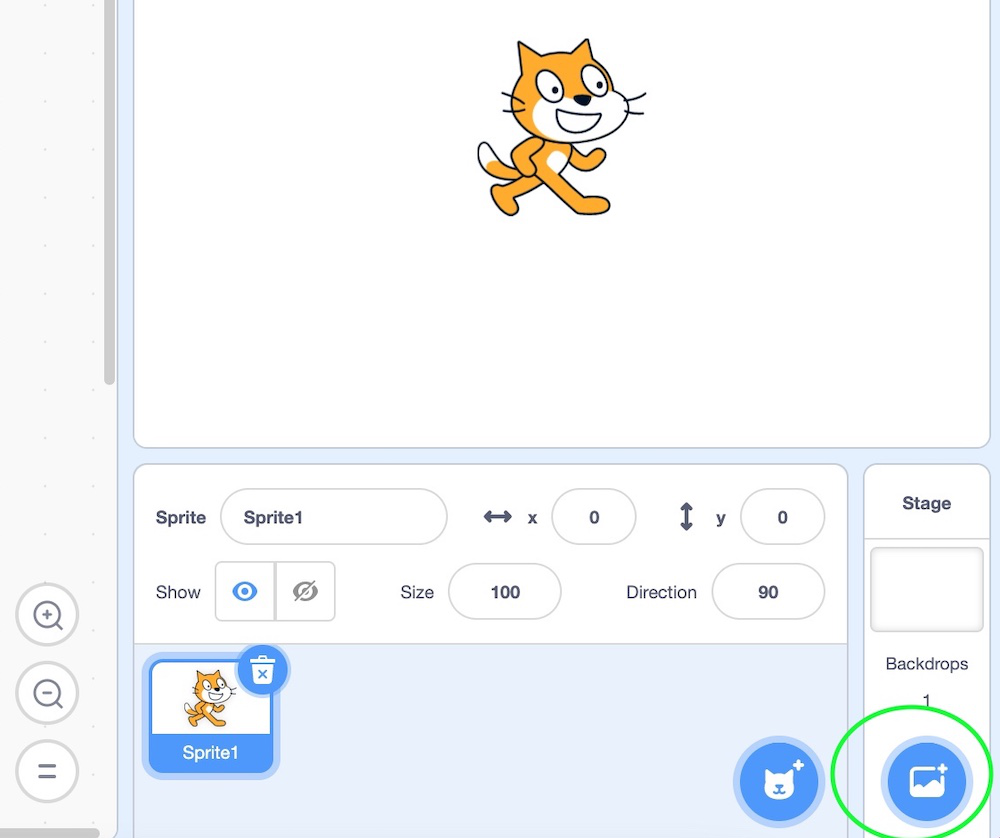There will be numerous lists of backdrops that scratch offers as shown below: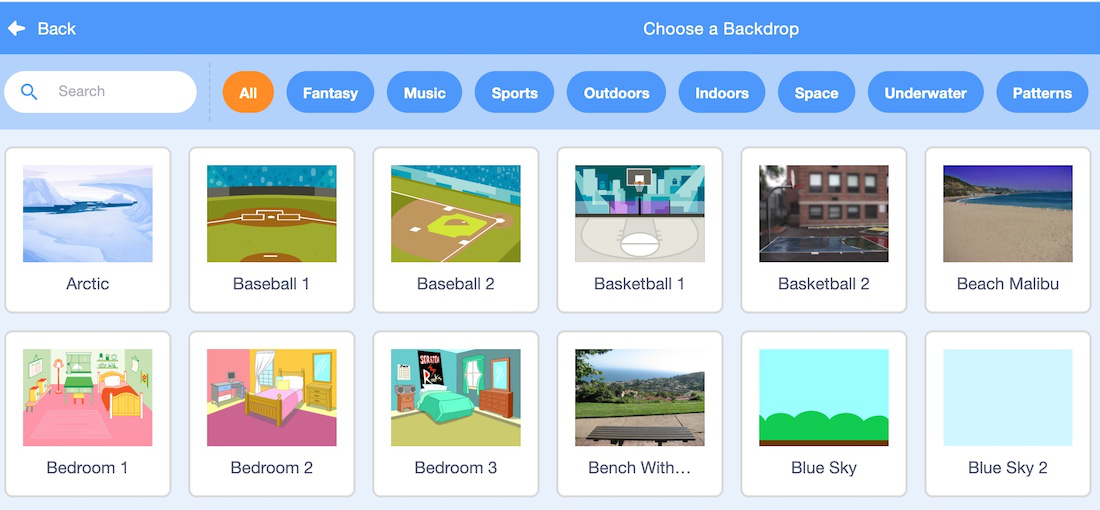Now, choose the “xy grid” backdrop and you will observe that the background of sprite changes to xy grid. This xy grid gives an exact idea of the minimum, maximum, and centre values of the x and y coordinate. When the sprite is moved from one place to another, the corresponding x, y coordinate is also shown simultaneously. This is depicted in the above gif.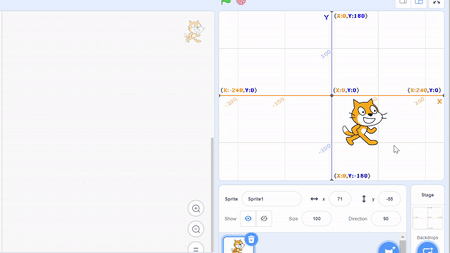The X position and Y position of a sprite can be determined, set, and changed by using the motion block. Below are the ways in which the coordinate of a sprite can be modified.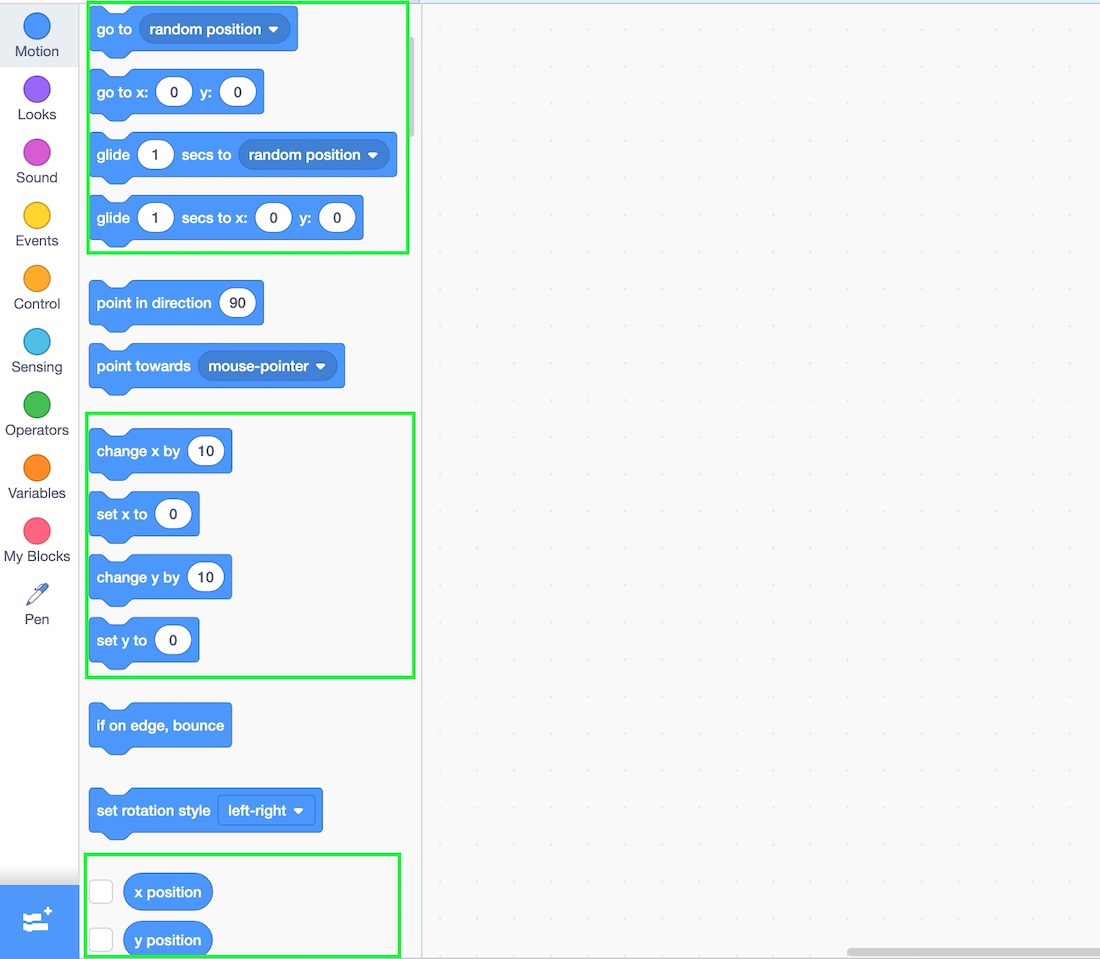### Conditionals in scratch

Conditional statements have conditions and the programs flow based on the true or false value of the condition. They are found in the control programming blocks as shown below.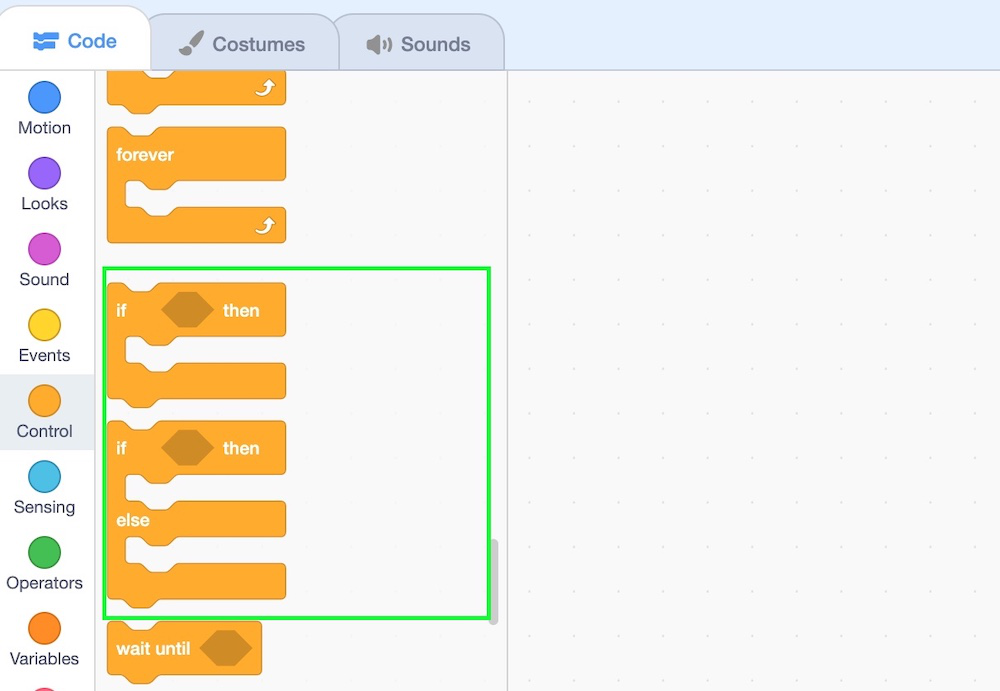Here, if() then and if() then else are the conditional blocks.

### 1. if() then block

It is a control block. This block works on the condition’s value. If the condition is true, then the set of code is executed else nothing happens. Here, the condition is only checked once, and if the condition is changed to false while the script is running, then the script will keep running until it is finished. It is just like the if-else statement in languages like Java, Python, etc. Let’s understand this concept by making a small project. Let us understand the working of the wait block with the help of a simple project. In this project, if the user types “a”, then the sprite must glide for 1 second.

Procedure:

Step 1: Click on event block and choose 1st control block denoting start of the program(drag it to center).

Step 2: Click on control block and drag if then block below the above step.

Step 3: Go to the sensing block and drag key space pressed and place it inside the condition of if-then block and change “space” to “a”

Step 4: Go to motion block and drag glide 1 sec to random position inside if-then block.

Step 5: Now, run the program.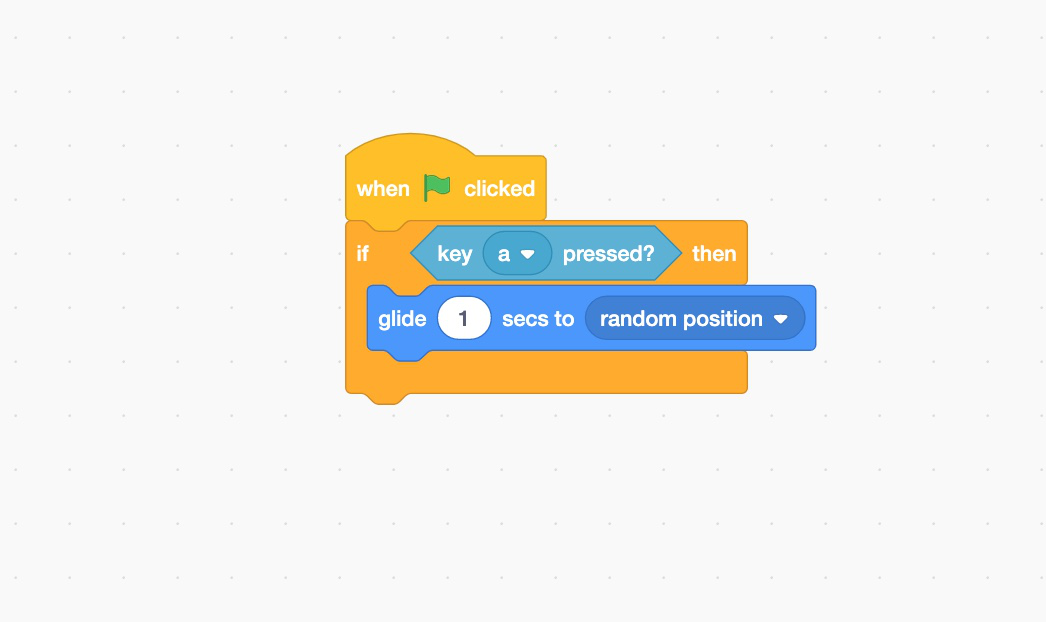Below is the implementation of the above procedure.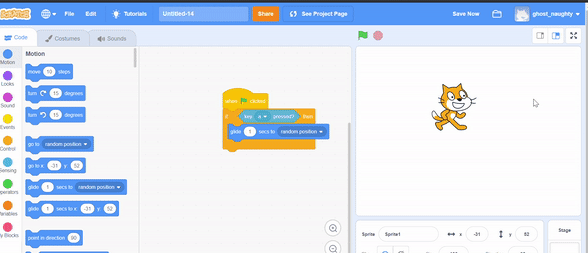### 2. if-then-else

It is a control block. This block is an extension of the if-then value. If a condition is true, then a set of lines gets executed. If a condition is false, then else part is executed. Let us understand the working of the wait block with the help of a simple project. In this project, if the user types “a”, then the sprite must glide for 1 second, else the sprite must say “hello”.

Procedure:

Step 1: Click on event block and choose 1st control block denoting the start of the program(drag it to center).

Step 2: Click on the control block and drag if then block below the above step.

Step 3: Go to the sensing block and drag key space pressed and place it inside the condition of if then block, change “space” to “a”

Step 4: Go to motion block and drag glide 1 sec to a random position, inside the if then block.

Step 5: Go to looks block and drag say hello block inside else part.

Step 6: Now run the program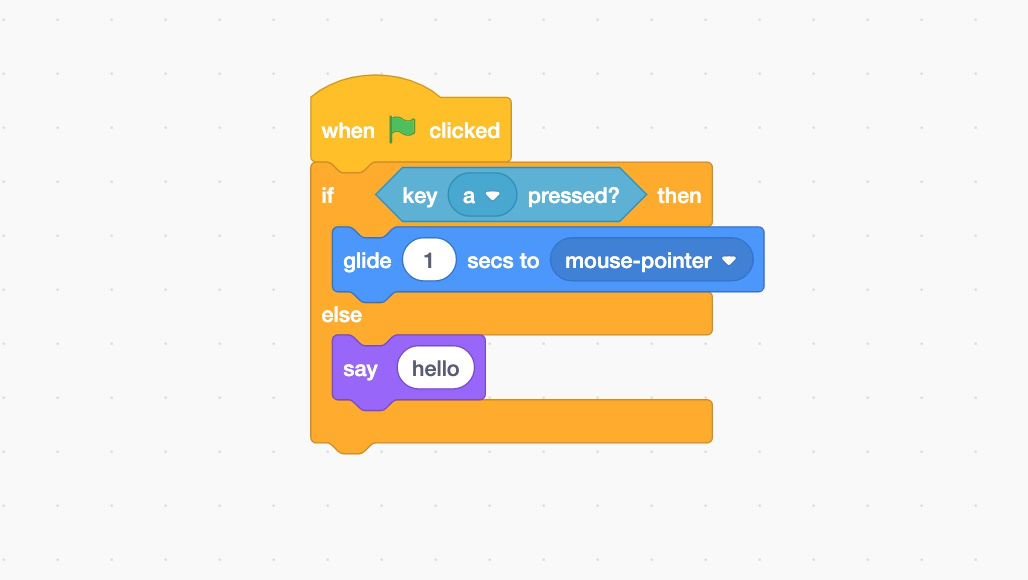Below is the implementation of the procedure: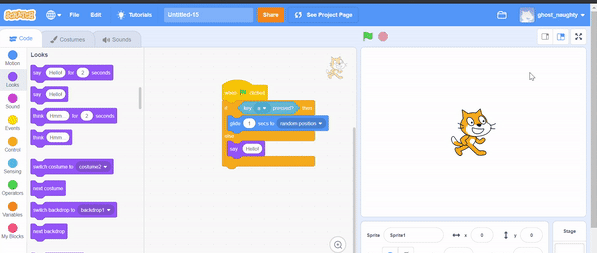My Personal Notes arrow_drop_up